# Hydrogen Peroxide

## Hydrogen Peroxide

Hydrogen peroxide is another hydride of oxygen. Unlike water it is highly unstable, and therefore, does not exist in nature as such. Its, importance, in recent years has increased due to its use as rocket fuel.

### Preparation of Hydrogen Peroxide

1. From sodium peroxide (Merck’s method)

Calculated amount of sodium peroxide (Na2O2) is gradually added to an ice-cold solution of 20% H2SO4   in small lots with constant stiring.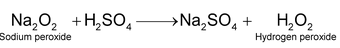Upon cooling, crystals of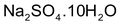separate out and the resulting solution contains about 30% H2O2. The solution also contains some dissolved Na2SO4, but it does not interfere with the reactions of H2O2. A pure sample of H2O2 may, however, be prepared by vacuum distillation.

2. From barium peroxide-Laboratory method of preparation

Hydrogen peroxide is prepared from barium peroxide by the following methods:

(a) By the action of dilute sulphuric acid. A paste of hydrated barium peroxide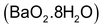is prepared in ice – cold water and then added slowly to an ice-cold solution of 20% H2SO4 .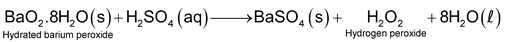The white precipitate of BaSO4 is removed by filtration leaving behind a dilute solution (5%) of H2O2. In this method, anhydrous barium peroxide can not used since the precipitated BaSO4 forms a protective layer around unreacted barium peroxide thereby preventing the further reaction

### Limitation

Hydrogen peroxide prepared by this method contains appreciable quantities of Ba2+ ions (in the form of dissolved barium persulphate) which catalyse the decomposition of H2O2.Therefore, H2O prepared by this method cannot be stored for a long time.

Further, H2SOalso acts as a catalyst for decomposition of H2O2.

(b) By the action of carbon dioxide or carbonic acid. When a rapid stream of CO2 is bubbled through a thin paste of BaO2 in ice-cold water, H2O2 and BaCO3 are produced: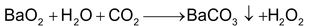The insoluble barium carbonate is removed by filtration leaving behind a dilute solution of  H2O2.

(c) By the action of phosphoric acid. Hydrogen peroxide can also be prepared by the action of phosphoric acid on barium peroxide: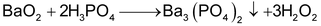This method has the advantage over BaO2- H2SO4 method since almost all the heavy metal (e.g. Pb etc) impurities present in BaO2 and which catalyse the decomposition of H2O2 are removed as insoluble phosphates. As a result, the resulting solution of H2O2 has good keeping properties.

### Manufacture of Hydrogen Peroxide

1. By electrolysis of 50% H2SO4

Hydrogen peroxide is manufactured by the electrolysis of a cold 50% solutions of H2SO4 in an electrolytic cell using platinum as anode and graphite as cathode. The reactions taking place are: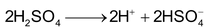At cathode: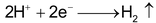At anode :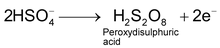Peroxydisulphuric acid formed around anode is withdrawn and then distilled with water under reduced pressure. The low boiling H2O2 distils over along with water leaving behind high boiling H2SO which is recovered and recycled.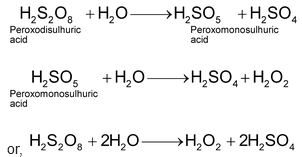### Modification

Recently, it has been observed that if instead of 50% H2SO an equimolar mixture of H2SO4 and ammonium sulphate is electrolysed, a more concentrated solution of H2O2 is obtained.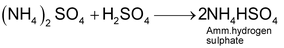At cathode:At Anode:

Ammonium persulphate formed around anode is withdrawn and distilled with water to give H2O2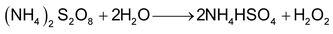This process is now used only for the laboratory preparation of D2O2, i.e.;

### Deutroperoxide

2. By autoxidation of 2-ethylanthraquinol

This is a new method and is widely used in U.S.A. In this process, air is bubbled through a 10% solution of 2-ethylanthraquinol in benzene and cyclohexane when 2-ethylanthraquinol is oxidized to 2-ethylanthraquinone and H2O2 is formed according to the following equations.

The H2O thus formed (about 1%) is extracted with water and the aqueous solution is concentrated by distillation under reduced pressure to give 30% (by weight) H2O2 solution.

2 – Ethylanthraquinone so produced can be reduced back to the starting to the material i.e., 2-ethylanthraquinol by hydrogen using palladium catalyst.

Thus, we find that the raw material are H2 and O2 only.

### Concentration of hydrogen Peroxide Solution

Hydrogen peroxide produced by any of the above method is in the form of dilute solution. The solution can not be concentrated by simple heating because it readily decomposes below its boiling point as

The dilute solution of hydrogen peroxide is concentrated carefully by the following steps:

(i) Slow evaporation on water bath

The dilute solution of hydrogen peroxide is taken in evaporating dish which is heated carefully on water bath. Slow evaporation continues causing the escaping of water molecules till approximately 50% solution of H2O2 is obtained.

(ii) Evaporation in vacuum desicator

The 50% solution is placed in vacuum desicator over concentrated sulphuric acid. The water vapours are absorbed by sulphuric acid leaving approximately 90% solution of H2O2.

(iii) Distillation under reduced pressure

The 90% solution of hydrogen peroxide is then subjected to distillation under reduced pressure (10 – 15mm). During this process, water distils over at 303 – 313 K and about 99% pure hydrogen peroxide is left behind.

(iv) Removal of last traces of water

The 99% solution of hydrogen peroxide is cooled in a freezing mixture of solid carbon dioxide and ether. As a result of this, crystals of hydrogen peroxide separate out which are removed, dried and remelted. This gives completely pure hydrogen peroxide.

## Storage of Hydrogen Peroxide

Decomposition of hydrogen peroxide into water oxygen is catalysed by traces of metal impurities, strong bases and exposure to light. Because of this property of H2O2, the concentrated solution of can be dangerous as uncontrolled rapid decomposition can result in an explosion. Thus,

(i) It is stored in wax lined coloured bottles as rough glass surface also causes its decomposition.

(ii) A small amount of stabilizer like phosphoric acid, or glycerol or acetanilide must to added to retard its decomposition.

Structure of Hydrogen Peroxide

As established by X – ray studies, hydrogen peroxide molecules has a non – planar structure. The molecules dimensions in gas phase and that in solid phase have given in figure. (a) and (b) respectively. In the crystal, the dihedral angle reduces to on account of hydrogen bonding. The two oxygen atoms are joined by a single electron – pair bond. The O – O Linkage is also called peroxide linkage.

### Properties of Hydrogen Peroxide

Some of the physical properties of hydrogen peroxide are listed below:

(i) Pure hydrogen peroxide is a colourless, syrupy liquid.

(ii) It has odour like that of nitric acid.

(iii) Its aqueous solution has a bitter taste.

(iv) It is soluble in water, alcohol and ether in all proportions.

(v) Its density is 1.44 g. The high density is due to association of its molecules by intermolecular hydrogen bonds.

(vi) Its boiling points is 423 K at 670 mm pressure and 358 K at 68 mm pressure. Its melting point is 272 K.

### Chemical Properties

(a) Decomposition

It is a unstable liquid readily decomposes on heating or on long standing to give water and dioxygen. It is an example of disproportionation decomposition is suppressed by addition of glycerol, acetanilide or phosphoric acid.

(b) Acidic behaviour

Pure hydrogen peroxide is a weak acid (at 298 K). it ionizes in water as:

Its acidic character can be shown its ability to neutralize bases such as NaOH, etc, to form corresponding peroxides

Hydrogen peroxide has an interesting chemistry because of its ability to act as oxidizing as well as reducing agent both in acidic and basic solutions.

Oxidising nature of H2O2

can acts as oxidizing agents in acidic as well as basic medium as described below.

### Bleaching Action

The bleaching action of hydrogen peroxide is due to the nascent oxygen which it liberates on decomposition.

The nascent oxygen combines with colouring matter which, in turn, gets oxidised. Thus, the bleaching action of is due to the oxidation of colouring matter by nascent oxygen. It is used for the bleaching of delicate materials like ivory, feather silk, wool etc.

Hydrogen peroxide reacts with alkenes to form glycols.

Strength of Hydrogen Peroxide

1. As percentage

Some time the actual concentration of hydrogen peroxide in a solution in expressed as percentages of in solution. Thus, 40% solution of hydrogen peroxide means 40 grams of hydrogen peroxide are present in 100 grams of solution.

2. As volume strength

The most common method of expressing the concentration of hydrogen peroxide solution is, in terms of the volume of oxygen which a solution of hydrogen peroxide gives on decomposition by heat. For example, “10 volume” hydrogen peroxide refers to a solution of hydrogen peroxide whose, 1 litre will give 10 litre of oxygen at STP.

### Calculation of Strength of H2O2

It is possible to calculate the strength of hydrogen peroxide from its “volume strength”. Let us calculate the concentration in g/litre of “30 volume” solution of hydrogen peroxide.

A 30 volume solution of hydrogen means that 1 litre of the solution gives 30 litre of oxygen at STP. According to its decomposition reaction.

Now 1 litre of hydrogen peroxide gives 30 L of oxygen at STP

From the above equation

22.4 L of oxygen is obtained from =

30 L of oxygen at STP is obtained from =

Thus, 1L of sample contains = 91.07g

or Strength of the 30 volume solution of hydrogen peroxide is 91.07